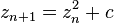Benoit B. Mandelbrot  (20 November 1924 – 14 October 2010) was a Polish-born, French and American mathematician, noted for developing a “theory of roughness” and “self-similarity” in nature and the field of fractal geometry to help prove it, which included coining the word “fractal”. He later discovered the Mandelbrot set of intricate, never-ending fractal shapes, named in his honor.

The Mandelbrot set is the set of complex numbers ‘c’ for which the sequence ( c, c² + c, (c²+c)² + c, ((c²+c)²+c)² + c, (((c²+c)²+c)²+c)² + c, …) does not approach infinity. The set is closely related to Julia sets (which include similarly complex shapes) and is named after the mathematician Benoit Mandelbrot, who studied and popularized it. Mandelbrot set images are made by sampling complex numbers and determining for each whether the result tends towards infinity when a particular mathematical operation is iterated on it. Treating the real and imaginary parts of each number as image coordinates, pixels are colored according to how rapidly the sequence diverges, if at all.

More precisely, the Mandelbrot set is the set of values of c in the complex plane for which the orbit of 0 underiteration of the complex quadratic polynomial$z_{n+1}=z_n^2+c$

remains bounded. That is, a complex number c is part of the Mandelbrot set if, when starting with z0 = 0 and applying the iteration repeatedly, the absolute value of zn remains bounded however large n gets.

For example, letting c = 1 gives the sequence 0, 1, 2, 5, 26,…, which tends to infinity. As this sequence is unbounded, 1 is not an element of the Mandelbrot set. On the other hand, c = −1 gives the sequence 0, −1, 0, −1, 0,…, which is bounded, and so −1 belongs to the Mandelbrot set.

Images of the Mandelbrot set display an elaborate boundary that reveals progressively ever-finer recursive detail at increasing magnifications. The “style” of this repeating detail depends on the region of the set being examined. The set’s boundary also incorporates smaller versions of the main shape, so the fractal property of self-similarity applies to the entire set, and not just to its parts.

The Mandelbrot set has become popular outside mathematics both for its aesthetic appeal and as an example of a complex structure arising from the application of simple rules, and is one of the best-known examples of mathematical visualization. (wikipedia)

This site uses Akismet to reduce spam. Learn how your comment data is processed.